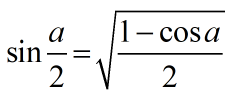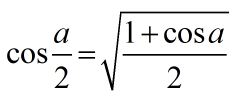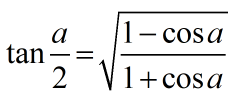Request a Tool

# Half Angle Identity Calculator

Half Angle Identity Calculator is use to calculate half angle of sin, cos and tan.

Half angle sin
0
Half angle sin
0

#### Formula• sin a/2 = Half angle of sin
• a = Value of angle

#### Defination / Uses

The sine and cosine half-angle identities are derived from two of the previously discussed cosine identities. The sign of the two preceding functions varies depending on which quadrant the resulting angle is in.

Half angle cos
0
Half angle cos
0

#### Formula• cos a/2 = Half angle of cos
• a = Value of angle

#### Defination / Uses

The sine and cosine half-angle identities are derived from two of the cosine identities stated above. The sign of the two previous functions is determined by the quadrant in which the resulting angle is found. In addition, 210° lies in the third quadrant, where cosine functions are negative.

Half angle tan
0
Half angle tan
0

#### Formula• tan a/2 = Half angle of tan
• a = Value of angle

#### Defination / Uses

Tangent half-angle formulae in trigonometry connect the tangent of half an angle to trigonometric functions of the complete angle. The stereographic projection of the circle onto a line is the tangent of half an angle.

We'll be studying half-angle trig identities on half-angle calculator. We can simply find the functions of twice the angle using the double angle formulas. We'd like to accomplish the same thing here, except instead of multiplying by two, we'll divide the angle. The power reducing identities are, in reality, the most important tool for finding the sin, cos, and tan half-angle formulas. Let's not get ahead of ourselves, though. There will be plenty of time in the next parts to go over everything carefully and thoroughly. It's time to get started, so make sure you're ready and awake!